This post deals with something niche but practical - getting ggplot2 to plot multiple fitted gaussians from a model with different amplitudes. Google failed to provide the answer I was looking for - if you can’t find it with Google, it must need a blog post.

We have a couple of different projects running at the moment that need us to think about mixture models - these are common in single molecule biophysics work as you frequently end up with two or more populations of molecules stretched out along a continuous variable; could be velocity, step size or something else. A common solution to figuring out what the typical representative values are in the mixed population is to fit multiple gaussians to the data and describe the mean and standard deviation of each. Doing this also allows you to describe the relative size of each population if this changes with conditions. The `mixtools` package1 for R does all this and more - it’s a fantastic resource (and the vignette2 is really well written too).

There are already some great blog posts3 4 that go into using the `normalmixEM` function to find a specified number (k) of best fit gaussians for your data. And also some that do a far better job than this biologist is ever going to do of explaining the Expectation-Maximization Algorithm5 that’s at work. It’s worth noting that `mixtools` has a whole suite of tools for estimating the number of gaussians in your sample (not trivial) and can deal with non-normal distributions.

### set up and getting the model

``````#libraries needed
library(tidyverse)
library(mixtools)

#just a plotting theme
ggplot2::theme_set(
theme_bw() +
theme(
plot.title = element_text(size = 14),
axis.title = element_text(size = 14, colour = "grey30"),
axis.text = element_text(size = 12, colour = "grey30"),
panel.grid = element_blank()
)
)``````

First let’s simulate a data set comprised of two groups with different means and the same standard deviation, but with half the observations in one group compared to the other:

``````set.seed(12) #better make this reproducible
observations <- tibble(value = c(
rnorm(n = 125, mean = 0.1, sd = 0.2), #the first normal distribution
rnorm(n = 250, mean = 0.8, sd = 0.2) #this second distribution is double the size of the first
)
)

#and a quick gander...
ggplot(observations, aes(x = value)) +
geom_histogram(binwidth = 0.05)``````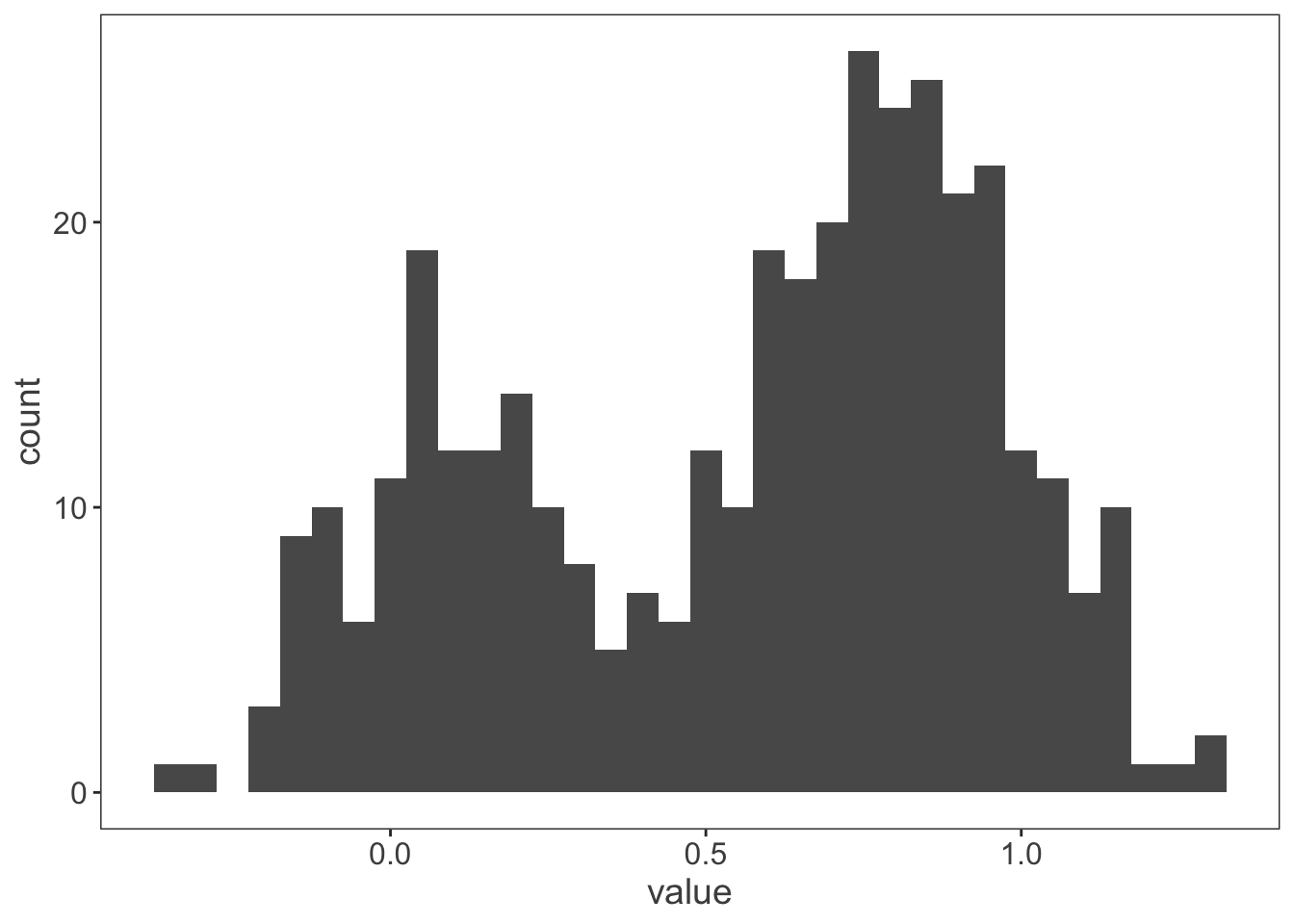Next let’s get `mixtools` to figure out where it thinks those gaussians are:

``my_mix <- normalmixEM(observations\$value, k = 2) #telling it to find two gaussians in the observations``
``## number of iterations= 38``
``plot(my_mix, which = 2) #looking at the model produced with base graphics``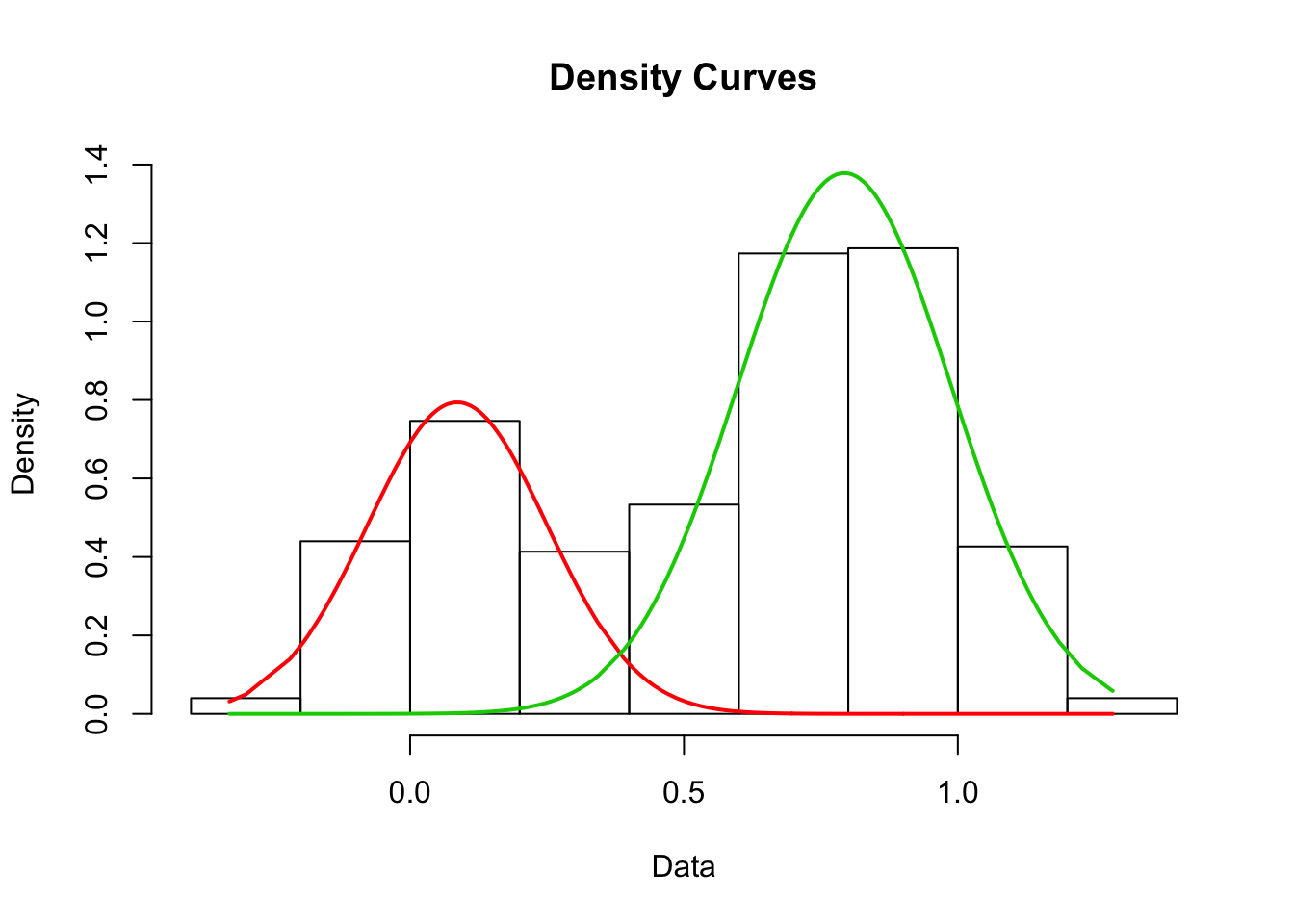### the problem

For publication ready plots we need to move over to ggplot2 rather than base graphics (some will argue no doubt, but there’s value in making graphics as human friendly as possible for communicating science). `normalmixEM` provides a list of 9 outputs for the gaussians it calculates, including the mean, standard deviation and amplitude:

``my_mix[["mu"]] #means``
``##  0.08612553 0.79291123``
``my_mix[["sigma"]] #standard deviations``
``##  0.1638908 0.1950395``
``my_mix[["lambda"]] #amplitudes``
``##  0.3262176 0.6737824``

It’s straight forward enough to use `dnorm` with `stat_function` to plot a gaussian line on top of a histogram. Two gaussians requires two `stat_functions` - not a big deal, but if you’ve got a data set with four or five, or you want to insert this more programmatically into an analysis script you quickly hit problems.

``````ggplot(observations, aes(x = value)) +
geom_histogram(binwidth = 0.05) +
stat_function(fun = dnorm,
args = list(mean = my_mix[["mu"]][],
sd = my_mix[["sigma"]][])) +
stat_function(fun = dnorm,
args = list(mean = my_mix[["mu"]][],
sd = my_mix[["sigma"]][]))``````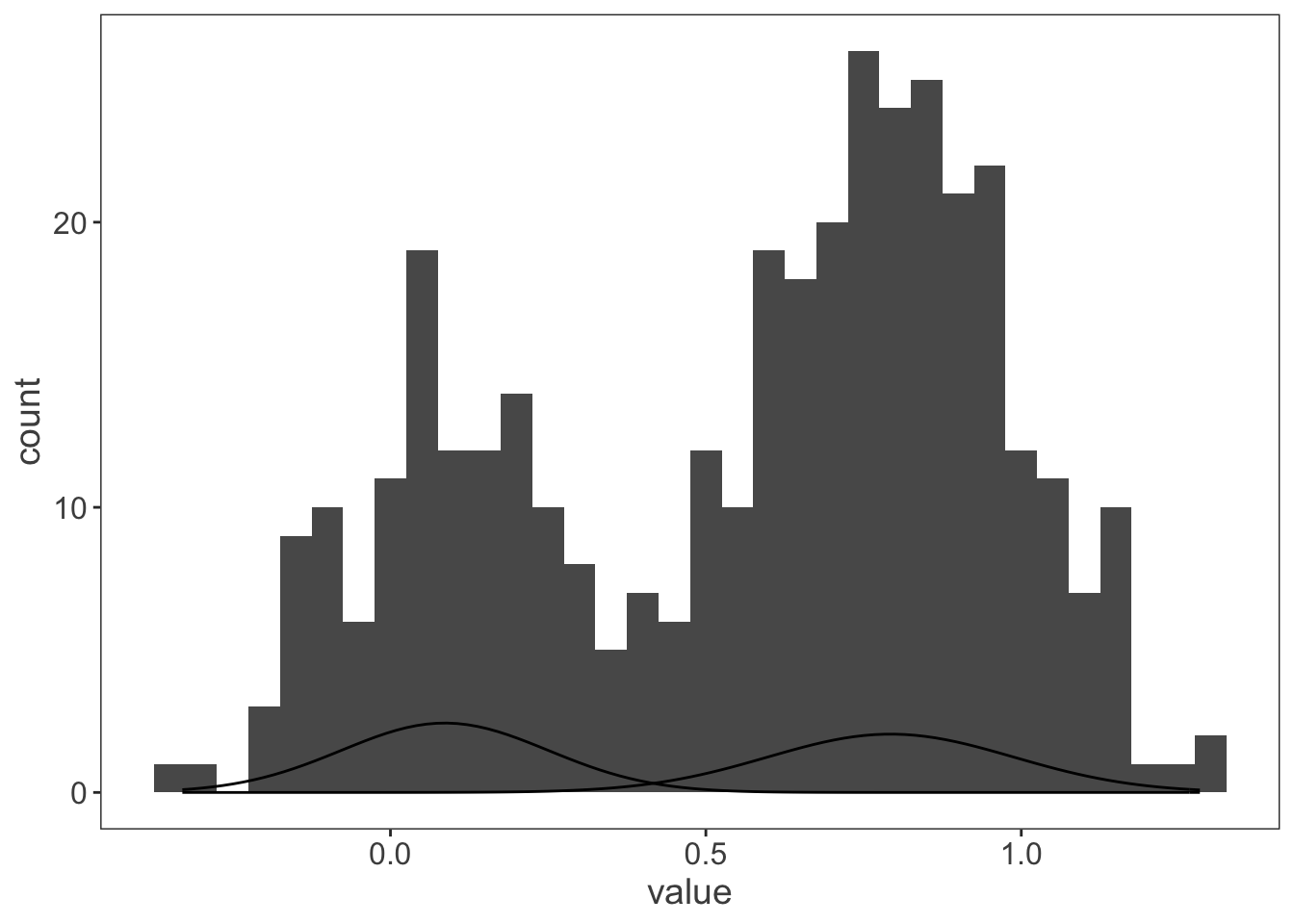There’s another couple of problems immediately. The gaussians are expressed as densities and the histogram as counts. Adding the aes `y = ..density..` to the histogram can fix this problem, but only if you want to express the data as a density instead of event counts. More difficult, `dnorm` doesn’t have an argument for the relative amplitude, so both gaussians have the same area. Three things need fixing - plot all the gaussians in a single call, convert them to be proportional to counts and incorporate the relative amplitude. Plotting multiple instances of a function requires `mapply` - the above plot can also be made like this:

``````ggplot(observations, aes(x = value)) +
geom_histogram(binwidth = 0.05) +
mapply(function(mean, sd) {
stat_function(fun = dnorm, args = list(mean = mean, sd = sd))
},
#the argument definitions hang out down here
mean = my_mix[["mu"]],
sd = my_mix[["sigma"]]
)``````### the solution

Scaling the gaussian density to the count number requires taking into account the number of observations in the data set and the bin width of the histogram. If we’re creating a function to modify `dnorm` then it can also incorporate the amplitude, `my_mix[["lambda"]]`. I need to nest a new function with extra arguments inside `stat_function` to modify `dnorm`.

``````ggplot(observations, aes(x = value)) +
geom_histogram(binwidth = 0.05) +
mapply(
function(mean, sd, lambda, n, binwidth) {
stat_function(
fun = function(x) {
(dnorm(x, mean = mean, sd = sd)) * n * binwidth * lambda
}
)
},
mean = my_mix[["mu"]], #mean
sd = my_mix[["sigma"]], #standard deviation
lambda = my_mix[["lambda"]], #amplitude
n = length(observations\$value), #sample size
binwidth = 0.05 #binwidth used for histogram
)``````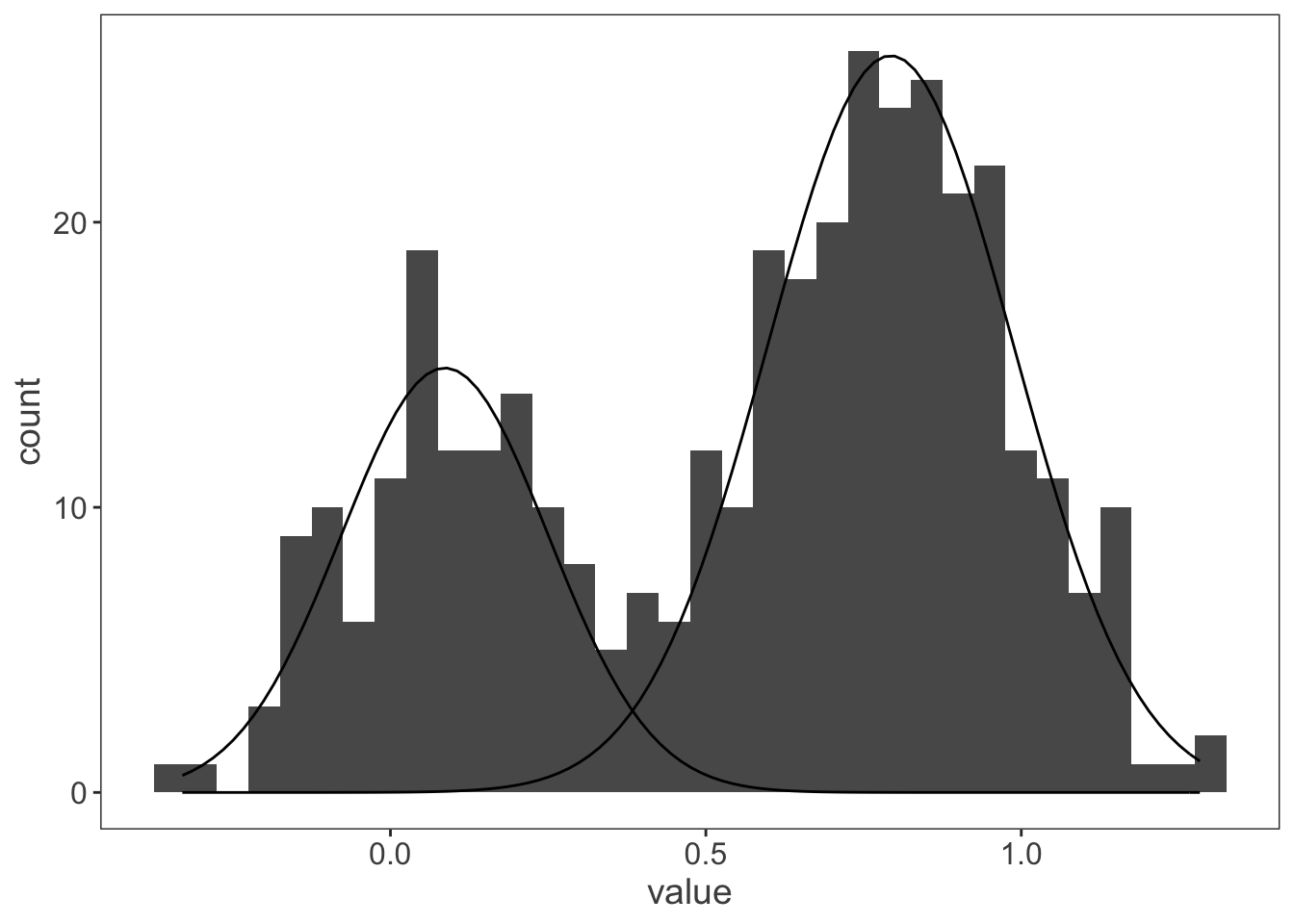That’s more like it! And just to check it works for a more complicated data set with more than two distributions:

``````observations <- tibble(value = c(
rnorm(n = 125, mean = 0.1, sd = 0.2),
rnorm(n = 175, mean = 0.4, sd = 0.05),
rnorm(n = 250, mean = 0.6, sd = 0.05),
rnorm(n = 250, mean = 0.8, sd = 0.2)
)
)

my_mix <- normalmixEM(observations\$value, k = 4)``````
``## number of iterations= 167``
``````ggplot(observations, aes(x = value)) +
geom_histogram(binwidth = 0.05) +
mapply(function(mean, sd, lambda, n, binwidth) {
stat_function(
fun = function(x) {
(dnorm(x, mean = mean, sd = sd)) * n * binwidth * lambda
}
)
},
mean = my_mix[["mu"]],
sd = my_mix[["sigma"]],
lambda = my_mix[["lambda"]],
n = length(observations\$value),
binwidth = 0.05
)``````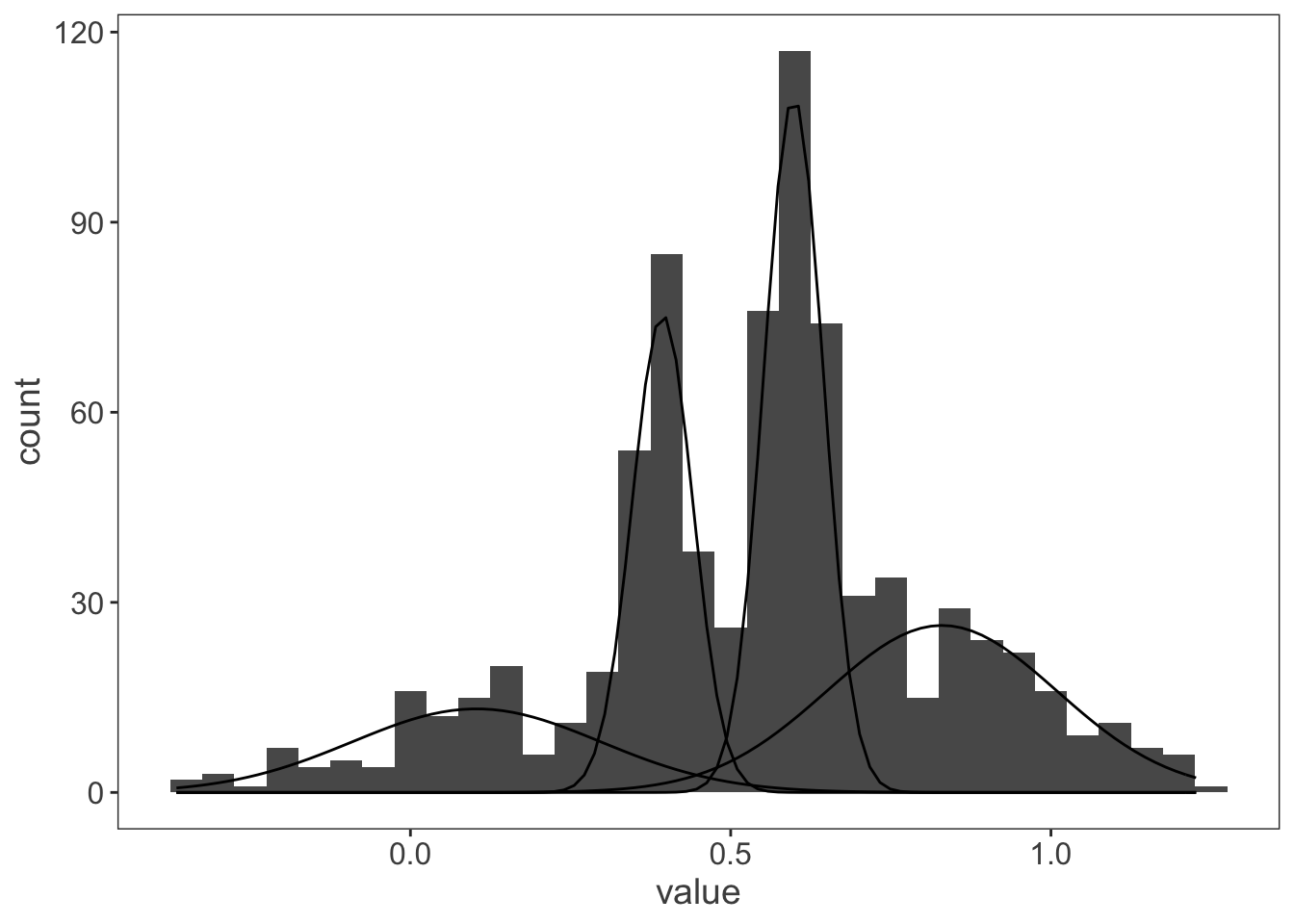### R session

``devtools::session_info()``
``````## ─ Session info ──────────────────────────────────────────────────────────
##  setting  value
##  version  R version 3.6.0 (2019-04-26)
##  os       macOS Mojave 10.14
##  system   x86_64, darwin15.6.0
##  ui       X11
##  language (EN)
##  collate  en_GB.UTF-8
##  ctype    en_GB.UTF-8
##  tz       Europe/London
##  date     2019-06-19
##
## ─ Packages ──────────────────────────────────────────────────────────────
##  package     * version  date       lib source
##  assertthat    0.2.1    2019-03-21  CRAN (R 3.6.0)
##  backports     1.1.4    2019-04-10  CRAN (R 3.6.0)
##  blogdown      0.13     2019-06-11  CRAN (R 3.6.0)
##  bookdown      0.11     2019-05-28  CRAN (R 3.6.0)
##  broom         0.5.2    2019-04-07  CRAN (R 3.6.0)
##  callr         3.2.0    2019-03-15  CRAN (R 3.6.0)
##  cellranger    1.1.0    2016-07-27  CRAN (R 3.6.0)
##  cli           1.1.0    2019-03-19  CRAN (R 3.6.0)
##  colorspace    1.4-1    2019-03-18  CRAN (R 3.6.0)
##  crayon        1.3.4    2017-09-16  CRAN (R 3.6.0)
##  desc          1.2.0    2018-05-01  CRAN (R 3.6.0)
##  devtools      2.0.2    2019-04-08  CRAN (R 3.6.0)
##  digest        0.6.19   2019-05-20  CRAN (R 3.6.0)
##  dplyr       * 0.8.1    2019-05-14  CRAN (R 3.6.0)
##  evaluate      0.14     2019-05-28  CRAN (R 3.6.0)
##  forcats     * 0.4.0    2019-02-17  CRAN (R 3.6.0)
##  fs            1.3.1    2019-05-06  CRAN (R 3.6.0)
##  generics      0.0.2    2018-11-29  CRAN (R 3.6.0)
##  ggplot2     * 3.1.1    2019-04-07  CRAN (R 3.6.0)
##  glue          1.3.1    2019-03-12  CRAN (R 3.6.0)
##  gtable        0.3.0    2019-03-25  CRAN (R 3.6.0)
##  haven         2.1.0    2019-02-19  CRAN (R 3.6.0)
##  hms           0.4.2    2018-03-10  CRAN (R 3.6.0)
##  htmltools     0.3.6    2017-04-28  CRAN (R 3.6.0)
##  httr          1.4.0    2018-12-11  CRAN (R 3.6.0)
##  jsonlite      1.6      2018-12-07  CRAN (R 3.6.0)
##  knitr         1.23     2019-05-18  CRAN (R 3.6.0)
##  labeling      0.3      2014-08-23  CRAN (R 3.6.0)
##  lattice       0.20-38  2018-11-04  CRAN (R 3.6.0)
##  lazyeval      0.2.2    2019-03-15  CRAN (R 3.6.0)
##  lubridate     1.7.4    2018-04-11  CRAN (R 3.6.0)
##  magrittr      1.5      2014-11-22  CRAN (R 3.6.0)
##  MASS          7.3-51.4 2019-03-31  CRAN (R 3.6.0)
##  Matrix        1.2-17   2019-03-22  CRAN (R 3.6.0)
##  memoise       1.1.0    2017-04-21  CRAN (R 3.6.0)
##  mixtools    * 1.1.0    2017-03-10  CRAN (R 3.6.0)
##  modelr        0.1.4    2019-02-18  CRAN (R 3.6.0)
##  munsell       0.5.0    2018-06-12  CRAN (R 3.6.0)
##  nlme          3.1-140  2019-05-12  CRAN (R 3.6.0)
##  pillar        1.4.1    2019-05-28  CRAN (R 3.6.0)
##  pkgbuild      1.0.3    2019-03-20  CRAN (R 3.6.0)
##  pkgconfig     2.0.2    2018-08-16  CRAN (R 3.6.0)
##  pkgload       1.0.2    2018-10-29  CRAN (R 3.6.0)
##  plyr          1.8.4    2016-06-08  CRAN (R 3.6.0)
##  prettyunits   1.0.2    2015-07-13  CRAN (R 3.6.0)
##  processx      3.3.1    2019-05-08  CRAN (R 3.6.0)
##  ps            1.3.0    2018-12-21  CRAN (R 3.6.0)
##  purrr       * 0.3.2    2019-03-15  CRAN (R 3.6.0)
##  R6            2.4.0    2019-02-14  CRAN (R 3.6.0)
##  Rcpp          1.0.1    2019-03-17  CRAN (R 3.6.0)
##  readr       * 1.3.1    2018-12-21  CRAN (R 3.6.0)
##  readxl        1.3.1    2019-03-13  CRAN (R 3.6.0)
##  remotes       2.0.4    2019-04-10  CRAN (R 3.6.0)
##  rlang         0.3.4    2019-04-07  CRAN (R 3.6.0)
##  rmarkdown     1.13     2019-05-22  CRAN (R 3.6.0)
##  rprojroot     1.3-2    2018-01-03  CRAN (R 3.6.0)
##  rstudioapi    0.10     2019-03-19  CRAN (R 3.6.0)
##  rvest         0.3.4    2019-05-15  CRAN (R 3.6.0)
##  scales        1.0.0    2018-08-09  CRAN (R 3.6.0)
##  segmented     0.5-4.0  2019-05-13  CRAN (R 3.6.0)
##  sessioninfo   1.1.1    2018-11-05  CRAN (R 3.6.0)
##  stringi       1.4.3    2019-03-12  CRAN (R 3.6.0)
##  stringr     * 1.4.0    2019-02-10  CRAN (R 3.6.0)
##  survival      2.44-1.1 2019-04-01  CRAN (R 3.6.0)
##  tibble      * 2.1.3    2019-06-06  CRAN (R 3.6.0)
##  tidyr       * 0.8.3    2019-03-01  CRAN (R 3.6.0)
##  tidyselect    0.2.5    2018-10-11  CRAN (R 3.6.0)
##  tidyverse   * 1.2.1    2017-11-14  CRAN (R 3.6.0)
##  usethis       1.5.0    2019-04-07  CRAN (R 3.6.0)
##  withr         2.1.2    2018-03-15  CRAN (R 3.6.0)
##  xfun          0.7      2019-05-14  CRAN (R 3.6.0)
##  xml2          1.2.0    2018-01-24  CRAN (R 3.6.0)
##  yaml          2.2.0    2018-07-25  CRAN (R 3.6.0)
##
##  /Library/Frameworks/R.framework/Versions/3.6/Resources/library``````